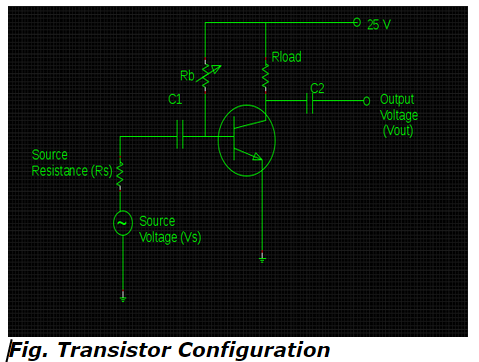Q1. It is feasible to draw DC load line of a transistor with an acquaintance of __________ points.

a. cut-off & saturation
b. active & cut-off
c. active & saturation
d. all of the above

Q2. Which point on the DC load line exhibit collector current and collector-emitter junction voltage that gets emerged in a transistor even without an application of input signal?

a. DC operating point
b. Quiescent Point
c. Working Point
d. All of the above

ANSWER: d. All of the above

Q3. What is the value of base resistance if the values of β & VBE are assumed to be 40 & 0.7 V respectively in order to obtain the operating point in the following schematic diagram ?a. 965 Ω
b. 972 Ω
c. 985 Ω
d. 962 Ω